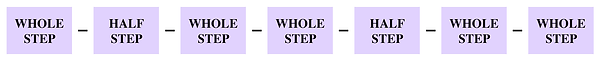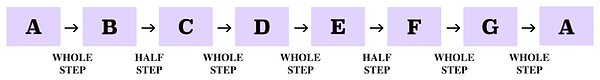top of page

## Mode Construction | Aeolian Mode (6 of 7)

In the first video of this series we learned how the Ionian mode is simply just another name for the notes of a Major scale. Well in that same respect, the notes of the Aeolian mode are exactly the same as the notes of a natural minor scale.

In other words, Aeolian mode is simply another name for the minor scale.

Just like the natural minor scale, the notes of the Aeolian mode may be constructed from the sixth scale degree of a Major scale.

Here are the notes of a C Major scale.Because the note A is the sixth scale degree, playing all of the notes in the key of C Major from the note A, to the next octave, a note also with the letter name A, would result in an A minor scale, otherwise known as A Aeolian.Let's also consider the notes of a G Major scale. We can see here that the sixth scale degree of a G Major scale is the note E.Therefore, if we played all of the notes in the key of G Major starting on the sixth scale degree, E, consecutively to the next note E, one octave above we will be playing E Aeolian.Now, as you probably guessed, the pattern of steps which constructs an Aeolian scale is exactly the same as the pattern of steps which constructs a natural minor scale.Let's use this pattern of steps to double check the notes of A Aeolian which we had constructed earlier in this video.Starting on the note A and going up one whole step takes us to the note B. A half step above the note B is the note C. A whole step separates the notes C and D. D to E is also a whole step. A half step separates the notes E and F. A whole step above the note F is the note G. And lastly, going up one whole step from the note G takes us to the note A.

By memorizing this pattern of steps you will be able to construct an Aeolian mode above any given note.